Antiques, Collectibles, Vintage Shopping
 Antique & Vintage Shop and Mall Collectics Speed Shopping Featured Sale Consignments Site Search
Collector Books, Reviews, Education
 Antiques & Collectibles Bookstore Collector Books Topic Search Collector Book Reviews Antique Price Guides Slideshow Antiques Information & Education
Online Museums & Directories
 Art Deco & Art Nouveau Museum Tiffany Lamps & Glass Museum Museum & Historic Site Directory Collecting & Design Directory
Discover Collectics Variety
Cool Stuff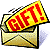Collectics Gift Certificates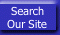Collectics Coupon Outlet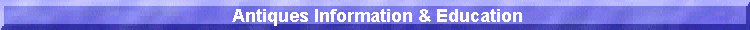Vintage Sunglasses Shop - Authentic vintage Revo, Ray-Ban, Armani, Carrera, Alpina, Cazal, Persol, Vuarnet, Bolle, Oliver Peoples, and French cat's eye sunglasses!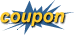Collectics Antique & Vintage Sale Coupon: Save 10% On Entire Order!

 The Collectics Antiques Information & Education pages are designed to further knowledge of antiques, collectibles, collecting styles, periods, artists, designers, and manufacturers of fine and decorative arts. To learn more, our Antique Collector Bookstore lists only the best collector books and price guides, complied by surveys of top antique dealers and auction houses. For a different shopping experience, you can also browse our featured selections in a fun new way with the Antique Price Guides Slideshow or see current Amazon.com bestsellers by using Collector Books Topic Search.
 The World of CollecticsLow Prices - High Quality: Antique & Vintage Shop!Antiques Information & Education Home PageBrowse Categories With Pictures: Speed Shopping!
 "prices 30% below your local antique shop or vintage store plus free shipping." Collectibles Guide 2010
 Peanuts © United Feature Syndicate, Inc.
ROMAN NUMERAL CONVERSION TABLE QUICK REFERENCE GUIDECollectics Customer Appreciation Sale Coupon: Save 10% On Entire Order!
 1500 -1549 1550 -1599 1600 -1649 1650 -1699 1700 -1749 1750 -1799 1800 -1849 1850 -1899 1900 -1949 1950 -1999 2000 -2049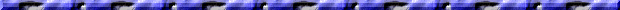1500 = MD 1501 = MDI 1502 = MDII 1503 = MDIII 1504 = MDIV 1505 = MDV 1506 = MDVI 1507 = MDVII 1508 = MDVIII 1509 = MDIX 1510 = MDX 1511 = MDXI 1512 = MDXII 1513 = MDXIII 1514 = MDXIV 1515 = MDXV 1516 = MDXVI 1517 = MDXVII 1518 = MDXVIII 1519 = MDXIX 1520 = MDXX 1521 = MDXXI 1522 = MDXXII 1523 = MDXXIII 1524 = MDXXIV 1525 = MDXXV 1526 = MDXXVI 1527 = MDXXVII 1528 = MDXXVIII 1529 = MDXXIX 1530 = MDXXX 1531 = MDXXXI 1532 = MDXXXII 1533 = MDXXXIII 1534 = MDXXXIV 1535 = MDXXXV 1536 = MDXXXVI 1537 = MDXXXVII 1538 = MDXXXVIII 1539 = MDXXXIX 1540 = MDXL 1541 = MDXLI 1542 = MDXLII 1543 = MDXLIII 1544 = MDXLIV 1545 = MDXLV 1546 = MDXLVI 1547 = MDXLVII 1548 = MDXLVIII 1549 = MDXLIX
 1550 = MDL 1551 = MDLI 1552 = MDLII 1553 = MDLIII 1554 = MDLIV 1555 = MDLV 1556 = MDLVI 1557 = MDLVII 1558 = MDLVIII 1559 = MDLIX 1560 = MDLX 1561 = MDLXI 1562 = MDLXII 1563 = MDLXIII 1564 = MDLXIV 1565 = MDLXV 1566 = MDLXVI 1567 = MDLXVII 1568 = MDLXVIII 1569 = MDLXIX 1570 = MDLXX 1571 = MDLXXI 1572 = MDLXXII 1573 = MDLXXIII 1574 = MDLXXIV 1575 = MDLXXV 1576 = MDLXXVI 1577 = MDLXXVII 1578 = MDLXXVIII 1579 = MDLXXIX 1580 = MDLXXX 1581 = MDLXXXI 1582 = MDLXXXII 1583 = MDLXXXIII 1584 = MDLXXXIV 1585 = MDLXXXV 1586 = MDLXXXVI 1587 = MDLXXXVII 1588 = MDLXXXVIII 1589 = MDLXXXIX 1590 = MDXC 1591 = MDXCI 1592 = MDXCII 1593 = MDXCIII 1594 = MDXCIV 1595 = MDXCV 1596 = MDXCVI 1597 = MDXCVII 1598 = MDXCVIII 1599 = MDXCIX
 1600 = MDC 1601 = MDCI 1602 = MDCII 1603 = MDCIII 1604 = MDCIV 1605 = MDCV 1606 = MDCVI 1607 = MDCVII 1608 = MDCVIII 1609 = MDCIX 1610 = MDCX 1611 = MDCXI 1612 = MDCXII 1613 = MDCXIII 1614 = MDCXIV 1615 = MDCXV 1616 = MDCXVI 1617 = MDCXVII 1618 = MDCXVIII 1619 = MDCXIX 1620 = MDCXX 1621 = MDCXXI 1622 = MDCXXII 1623 = MDCXXIII 1624 = MDCXXIV 1625 = MDCXXV 1626 = MDCXXVI 1627 = MDCXXVII 1628 = MDCXXVIII 1629 = MDCXXIX 1630 = MDCXXX 1631 = MDCXXXI 1632 = MDCXXXII 1633 = MDCXXXIII 1634 = MDCXXXIV 1635 = MDCXXXV 1636 = MDCXXXVI 1637 = MDCXXXVII 1638 = MDCXXXVIII 1639 = MDCXXXIX 1640 = MDCXL 1641 = MDCXLI 1642 = MDCXLII 1643 = MDCXLIII 1644 = MDCXLIV 1645 = MDCXLV 1646 = MDCXLVI 1647 = MDCXLVII 1648 = MDCXLVIII 1649 = MDCXLIX
 1650 = MDCL 1651 = MDCLI 1652 = MDCLII 1653 = MDCLIII 1654 = MDCLIV 1655 = MDCLV 1656 = MDCLVI 1657 = MDCLVII 1658 = MDCLVIII 1659 = MDCLIX 1660 = MDCLX 1661 = MDCLXI 1662 = MDCLXII 1663 = MDCLXIII 1664 = MDCLXIV 1665 = MDCLXV 1666 = MDCLXVI 1667 = MDCLXVII 1668 = MDCLXVIII 1669 = MDCLXIX 1670 = MDCLXX 1671 = MDCLXXI 1672 = MDCLXXII 1673 = MDCLXXIII 1674 = MDCLXXIV 1675 = MDCLXXV 1676 = MDCLXXVI 1677 = MDCLXXVII 1678 = MDCLXXVIII 1679 = MDCLXXIX 1680 = MDCLXXX 1681 = MDCLXXXI 1682 = MDCLXXXII 1683 = MDCLXXXIII 1684 = MDCLXXXIV 1685 = MDCLXXXV 1686 = MDCLXXXVI 1687 = MDCLXXXVII 1688 = MDCLXXXVIII 1689 = MDCLXXXIX 1690 = MDCXC 1691 = MDCXCI 1692 = MDCXCII 1693 = MDCXCIII 1694 = MDCXCIV 1695 = MDCXCV 1696 = MDCXCVI 1697 = MDCXCVII 1698 = MDCXCVIII 1699 = MDCXCIX
 1700 = MDCC 1701 = MDCCI 1702 = MDCCII 1703 = MDCCIII 1704 = MDCCIV 1705 = MDCCV 1706 = MDCCVI 1707 = MDCCVII 1708 = MDCCVIII 1709 = MDCCIX 1710 = MDCCX 1711 = MDCCXI 1712 = MDCCXII 1713 = MDCCXIII 1714 = MDCCXIV 1715 = MDCCXV 1716 = MDCCXVI 1717 = MDCCXVII 1718 = MDCCXVIII 1719 = MDCCXIX 1720 = MDCCXX 1721 = MDCCXXI 1722 = MDCCXXII 1723 = MDCCXXIII 1724 = MDCCXXIV 1725 = MDCCXXV 1726 = MDCCXXVI 1727 = MDCCXXVII 1728 = MDCCXXVIII 1729 = MDCCXXIX 1730 = MDCCXXX 1731 = MDCCXXXI 1732 = MDCCXXXII 1733 = MDCCXXXIII 1734 = MDCCXXXIV 1735 = MDCCXXXV 1736 = MDCCXXXVI 1737 = MDCCXXXVII 1738 = MDCCXXXVIII 1739 = MDCCXXXIX 1740 = MDCCXL 1741 = MDCCXLI 1742 = MDCCXLII 1743 = MDCCXLIII 1744 = MDCCXLIV 1745 = MDCCXLV 1746 = MDCCXLVI 1747 = MDCCXLVII 1748 = MDCCXLVIII 1749 = MDCCXLIX
 1750 = MDCCL 1751 = MDCCLI 1752 = MDCCLII 1753 = MDCCLIII 1754 = MDCCLIV 1755 = MDCCLV 1756 = MDCCLVI 1757 = MDCCLVII 1758 = MDCCLVIII 1759 = MDCCLIX 1760 = MDCCLX 1761 = MDCCLXI 1762 = MDCCLXII 1763 = MDCCLXIII 1764 = MDCCLXIV 1765 = MDCCLXV 1766 = MDCCLXVI 1767 = MDCCLXVII 1768 = MDCCLXVIII 1769 = MDCCLXIX 1770 = MDCCLXX 1771 = MDCCLXXI 1772 = MDCCLXXII 1773 = MDCCLXXIII 1774 = MDCCLXXIV 1775 = MDCCLXXV 1776 = MDCCLXXVI 1777 = MDCCLXXVII 1778 = MDCCLXXVIII 1779 = MDCCLXXIX 1780 = MDCCLXXX 1781 = MDCCLXXXI 1782 = MDCCLXXXII 1783 = MDCCLXXXIII 1784 = MDCCLXXXIV 1785 = MDCCLXXXV 1786 = MDCCLXXXVI 1787 = MDCCLXXXVII 1788 = MDCCLXXXVIII 1789 = MDCCLXXXIX 1790 = MDCCXC 1791 = MDCCXCI 1792 = MDCCXCII 1793 = MDCCXCIII 1794 = MDCCXCIV 1795 = MDCCXCV 1796 = MDCCXCVI 1797 = MDCCXCVII 1798 = MDCCXCVIII 1799 = MDCCXCIX
 1800 = MDCCC 1801 = MDCCCI 1802 = MDCCCII 1803 = MDCCCIII 1804 = MDCCCIV 1805 = MDCCCV 1806 = MDCCCVI 1807 = MDCCCVII 1808 = MDCCCVIII 1809 = MDCCCIX 1810 = MDCCCX 1811 = MDCCCXI 1812 = MDCCCXII 1813 = MDCCCXIII 1814 = MDCCCXIV 1815 = MDCCCXV 1816 = MDCCCXVI 1817 = MDCCCXVII 1818 = MDCCCXVIII 1819 = MDCCCXIX 1820 = MDCCCXX 1821 = MDCCCXXI 1822 = MDCCCXXII 1823 = MDCCCXXIII 1824 = MDCCCXXIV 1825 = MDCCCXXV 1826 = MDCCCXXVI 1827 = MDCCCXXVII 1828 = MDCCCXXVIII 1829 = MDCCCXXIX 1830 = MDCCCXXX 1831 = MDCCCXXXI 1832 = MDCCCXXXII 1833 = MDCCCXXXIII 1834 = MDCCCXXXIV 1835 = MDCCCXXXV 1836 = MDCCCXXXVI 1837 = MDCCCXXXVII 1838 = MDCCCXXXVIII 1839 = MDCCCXXXIX 1840 = MDCCCXL 1841 = MDCCCXLI 1842 = MDCCCXLII 1843 = MDCCCXLIII 1844 = MDCCCXLIV 1845 = MDCCCXLV 1846 = MDCCCXLVI 1847 = MDCCCXLVII 1848 = MDCCCXLVIII 1849 = MDCCCXLIX
 1850 = MDCCCL 1851 = MDCCCLI 1852 = MDCCCLII 1853 = MDCCCLIII 1854 = MDCCCLIV 1855 = MDCCCLV 1856 = MDCCCLVI 1857 = MDCCCLVII 1858 = MDCCCLVIII 1859 = MDCCCLIX 1860 = MDCCCLX 1861 = MDCCCLXI 1862 = MDCCCLXII 1863 = MDCCCLXIII 1864 = MDCCCLXIV 1865 = MDCCCLXV 1866 = MDCCCLXVI 1867 = MDCCCLXVII 1868 = MDCCCLXVIII 1869 = MDCCCLXIX 1870 = MDCCCLXX 1871 = MDCCCLXXI 1872 = MDCCCLXXII 1873 = MDCCCLXXIII 1874 = MDCCCLXXIV 1875 = MDCCCLXXV 1876 = MDCCCLXXVI 1877 = MDCCCLXXVII 1878 = MDCCCLXXVIII 1879 = MDCCCLXXIX 1880 = MDCCCLXXX 1881 = MDCCCLXXXI 1882 = MDCCCLXXXII 1883 = MDCCCLXXXIII 1884 = MDCCCLXXXIV 1885 = MDCCCLXXXV 1886 = MDCCCLXXXVI 1887 = MDCCCLXXXVII 1888 = MDCCCLXXXVIII 1889 = MDCCCLXXXIX 1890 = MDCCCXC 1891 = MDCCCXCI 1892 = MDCCCXCII 1893 = MDCCCXCIII 1894 = MDCCCXCIV 1895 = MDCCCXCV 1896 = MDCCCXCVI 1897 = MDCCCXCVII 1898 = MDCCCXCVIII 1899 = MDCCCXCIX
 1900 = MCM 1901 = MCMI 1902 = MCMII 1903 = MCMIII 1904 = MCMIV 1905 = MCMV 1906 = MCMVI 1907 = MCMVII 1908 = MCMVIII 1909 = MCMIX 1910 = MCMX 1911 = MCMXI 1912 = MCMXII 1913 = MCMXIII 1914 = MCMXIV 1915 = MCMXV 1916 = MCMXVI 1917 = MCMXVII 1918 = MCMXVIII 1919 = MCMXIX 1920 = MCMXX 1921 = MCMXXI 1922 = MCMXXII 1923 = MCMXXIII 1924 = MCMXXIV 1925 = MCMXXV 1926 = MCMXXVI 1927 = MCMXXVII 1928 = MCMXXVIII 1929 = MCMXXIX 1930 = MCMXXX 1931 = MCMXXXI 1932 = MCMXXXII 1933 = MCMXXXIII 1934 = MCMXXXIV 1935 = MCMXXXV 1936 = MCMXXXVI 1937 = MCMXXXVII 1938 = MCMXXXVIII 1939 = MCMXXXIX 1940 = MCMXL 1941 = MCMXLI 1942 = MCMXLII 1943 = MCMXLIII 1944 = MCMXLIV 1945 = MCMXLV 1946 = MCMXLVI 1947 = MCMXLVII 1948 = MCMXLVIII 1949 = MCMXLIX
 1950 = MCML 1951 = MCMLI 1952 = MCMLII 1953 = MCMLIII 1954 = MCMLIV 1955 = MCMLV 1956 = MCMLVI 1957 = MCMLVII 1958 = MCMLVIII 1959 = MCMLIX 1960 = MCMLX 1961 = MCMLXI 1962 = MCMLXII 1963 = MCMLXIII 1964 = MCMLXIV 1965 = MCMLXV 1966 = MCMLXVI 1967 = MCMLXVII 1968 = MCMLXVIII 1969 = MCMLXIX 1970 = MCMLXX 1971 = MCMLXXI 1972 = MCMLXXII 1973 = MCMLXXIII 1974 = MCMLXXIV 1975 = MCMLXXV 1976 = MCMLXXVI 1977 = MCMLXXVII 1978 = MCMLXXVIII 1979 = MCMLXXIX 1980 = MCMLXXX 1981 = MCMLXXXI 1982 = MCMLXXXII 1983 = MCMLXXXIII 1984 = MCMLXXXIV 1985 = MCMLXXXV 1986 = MCMLXXXVI 1987 = MCMLXXXVII 1988 = MCMLXXXVIII 1989 = MCMLXXXIX 1990 = MCMXC 1991 = MCMXCI 1992 = MCMXCII 1993 = MCMXCIII 1994 = MCMXCIV 1995 = MCMXCV 1996 = MCMXCVI 1997 = MCMXCVII 1998 = MCMXCVIII 1999 = MCMXCIX
 2000 = MM 2001 = MMI 2002 = MMII 2003 = MMIII 2004 = MMIV 2005 = MMV 2006 = MMVI 2007 = MMVII 2008 = MMVIII 2009 = MMIX 2010 = MMX 2011 = MMXI 2012 = MMXII 2013 = MMXIII 2014 = MMXIV 2015 = MMXV 2016 = MMXVI 2017 = MMXVII 2018 = MMXVIII 2019 = MMXIX 2020 = MMXX 2021 = MMXXI 2022 = MMXXII 2023 = MMXXIII 2024 = MMXXIV 2025 = MMXXV 2026 = MMXXVI 2027 = MMXXVII 2028 = MMXXVIII 2029 = MMXXIX 2030 = MMXXX 2031 = MMXXXI 2032 = MMXXXII 2033 = MMXXXIII 2034 = MMXXXIV 2035 = MMXXXV 2036 = MMXXXVI 2037 = MMXXXVII 2038 = MMXXXVIII 2039 = MMXXXIX 2040 = MMXL 2041 = MMXLI 2042 = MMXLII 2043 = MMXLIII 2044 = MMXLIV 2045 = MMXLV 2046 = MMXLVI 2047 = MMXLVII 2048 = MMXLVIII 2049 = MMXLIX

 Ever been fooled by a fake or a seller that didn't deliver the goods as described? At Collectics, we authenticate and stand behind everything we sell, at prices "30% below your local antique shop" according to Collectibles Guide 2010. Please browse our main Antiques & Collectibles Mall to find a treat for yourself or a great gift for others, all with free shipping. Thanks for visiting and shopping at Collectics!

Use our convenient Search Engine to find collecting and gift ideas from our ever changing diversity of finer antiques & collectibles!Collectics Antiques & Collectibles Collector Bookstore Only the best collector books and price guides on collecting antiques and collectibles, plus Amazon.com Topic Search & top rated Collector Book Reviews!Frederick Carder and Steuben Glass: American Classics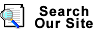Rookwood Pottery: The Glaze Lines With Value Guide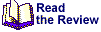Art Deco: 1910-1939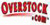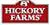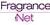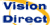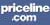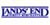Cool Coupons & Promo Coupon Codes for Disney, Brookstone, Avon, Netflix, eBags, and more @ our Coupon Site!
 Click On Collector Book Price Guides For Details Or Visit Our Antiques & Collectibles Bookstore
 Search Now:Amazon.com: Earth's Biggest Store!The Lamps of Tiffany
 Collector Book Purchases & Advertising Support Our Free Online Museums, Antiques & Collectibles Information, and Directories - Thank You!
 Schiffer Books For Collectors: Collector books and price guides by top antique appraisers & experts!Display Cases for collectibles, figurines, diecast cars, sports memorabilia, dolls, and more!